# 汽车毫米波雷达距离测量中的一种扩展卡尔曼滤波实现

2020-02-25 来源：微波射频网作者：安富利-王洪胜、王尧青、张秋英 我要评论(0) 字号：

1、卡尔曼滤波介绍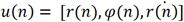(1)

r(n)代表目标相对于雷达的径向距离，φ(n)代表目标相对于雷达角度，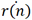代表目标相对于雷达径向速度。由于毫米波雷达测量得到的距离速度等信息都是径向的，因此测量向量u(n)是在极坐标系下获得的。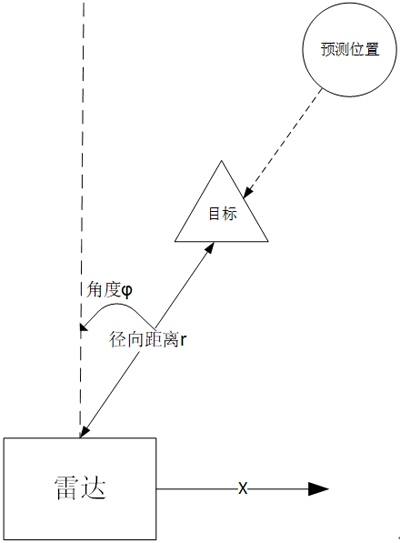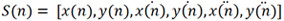(2)(3)

Sapr (n)=F*S(n-1)    (4)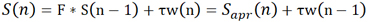(5)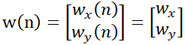(6)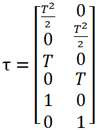(7)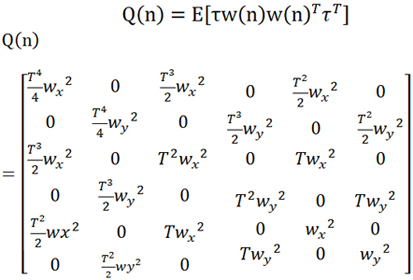(8)

Sapr (n)中完全不含有τw(n-1)导致的状态变化，由于无法准确量化w(n-1)，也可以理解S(n)始终不能完全涵盖τw(n-1)，实际上S(n)中还未考虑状态向量S(n-1)中缺失的信息，我们定义S(n)中缺少的信息总和为d(n)，由于同样无法准确计算d(n)，我们期望通过递归收敛的方法解决d(n)。定义d(n)的协方差矩阵为P(n)=E[d(n) d(n)T ]，P(n)矩阵大小为6*6。假设我们已知P(n-1)，那么可以推算出Papr (n)，公式如下：

Papr (n)=F*P(n-1)*FT+Q(n-1)    (9)

F*P(n-1)* FT可以理解为S(n-1)状态缺少的信息通过转移矩阵F到状态S(n)时产生的缺少信息的协方差矩阵，Q(n-1)为外力在时刻(n-1)T到时刻nT时间内引入的状态协方差矩阵。

u(n)=H(Sapr (n) )+JH (Sapr (n) )[S(n)-Sapr (n) ]+v(n)    (10)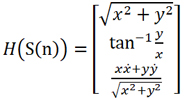(11)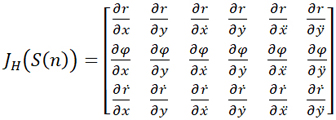(12)(13)(14)

S(n)=Sapr (n)+K(n)y(n)   (15)

K(n)y(n)是根据卡尔曼增益，从y(n)中得到的缺失信息，由于y(n)中既混合测量噪声又包括缺失信息，因此并不能从K(n)y(n)中获得缺失信息并屏蔽测量噪声，实际上缺失信息和测量噪声按照同样的比例关系（卡尔曼增益）被更新到状态向量S(n)中。所以S(n)中混合了部分测量噪声而且仍然是信息缺失的，缺失的信息更新如下：

P(n)=Papr (n)-K(n)JH (Sapr (n))Papr (n)   (16)

K(n)JH (Sapr (n))Papr (n)代表S(n)中已经通过K(n)y(n)获得信息，那么P(n)就代表状态向量S(n)中残留缺失信息，用于下一次迭代。也可以认为我们通过卡尔曼滤波器从新获得了部分缺失信息K(n)JH (Sapr (n))Papr (n)，更新后的P(n)代表S(n)尚缺少的信息，将被用于S(n+1)次的状态迭代。

2、实践中的修正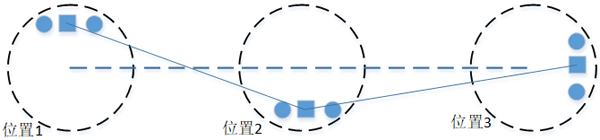3、雷达简介

 参数 数值 载波频率（Hz） 7.70E+10 载波波长（米） 3.90E-03 采样间隔（秒） 5.00E-08 啁啾周期（秒） 4.50E-05 啁啾采样周期（秒） 2.56E-05 单啁啾采样点数 512 啁啾个数 128 扫频带宽（Hz） 2.00E+08 距离分辨率（米） 7.50E-01 速度分辨率（米/秒） 3.38E-01 无模糊最大速度（米/秒） 2.16E+01 固定单元格最大速度（米/秒） 6.51E+01 无模糊最大距离（米） 1.92E+02

4、仿真验证5、结论

6、参考

 微波射频行业人士 | 相聚在这里【10大细分领域的微信技术交流群】订阅微信公众号加群管理员为好友